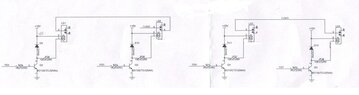# Understanding this Circuit involving Relays

• PhysicsTest

#### PhysicsTest

TL;DR Summary
I need to understand the hardware circuit.
I need to understand the below circuit, the RB4, RB5, RB6, RB7 are controlled by the micro controller. The COM2 and COM3 are going as input to the micro. I know when the outputs RB4 to RB7 are ON, the relays will be ON and 3,4 get shorted. But i don't understand the purpose the circuit. Am i sorry that i don't have much details. Any comments can help me, I heard it can be used to change star to delta connection and vice versa.Just to add this is the circuit used for phase voltage, i need to understand. Can I assume that the calculations for Y phase voltage at the micro input is 560K and 560K in series and 10K in parallel
Hence for 100V for example
Voltage at micro = (100*10)/(560K + 560K + 10K) = 0.97V. Please advise, i am not going to design this circuit, this is only for understanding purpose.

#### Attachments

Voltage at micro = (100*10)/(560K + 560K + 10K) = 0.97V.
Check your arithmetic and the missing k multiplier ?
100V *( 10k / ( 10k + 560k + 560k ) ) = 0.885 V

But i don't understand the purpose the circuit. Am i sorry that i don't have much details.
The text is important. There is too much white space. Do you have a readable schematic ?